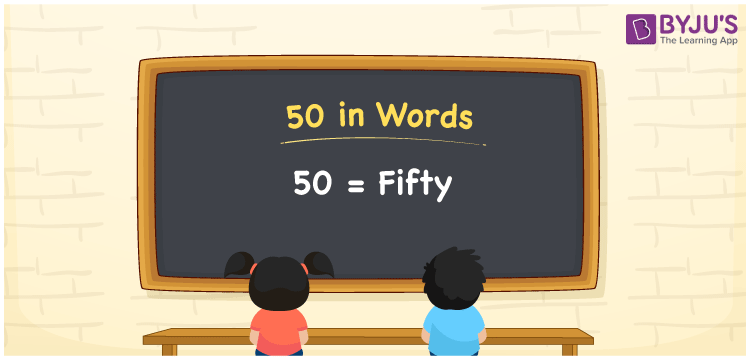# 50 in words

We can write 50 in words as Fifty. Suppose you bought a new book that costs Rs. 50, then you could say that “ I bought a new pen for fifty rupees”. In this article, you will learn how to convert the number 50 into words in an exciting way.

 50 in words Fifty Fifty in Numbers 50

## 50 in English Words

We generally write numbers in words using the English alphabet. Thus, we can read 50 in English as “Fifty”.## How to Write 50 in Words?

In this section, you will learn how to write the number 50 in words. The given number 50 has two digits, so we should make a place value chart that represents the place value for all these two digits. The order of place values of digits in a number is in accordance with the Indian numbering system, such as:

• Ones
• Tens
• Hundreds
• Thousands
• Ten-thousands
• Hundred-thousands or Lakhs

This can be done as follows.

 Tens Ones 5 0

This can be written as:

5 × Ten + 0 × One

= 5 × 10 + 0 × 1

= 50 + 0

= 50

= Fifty

Thus, 50 in words is written as Fifty.

50 is a natural number that precedes 51 and succeeds 49.

50 in words – Fifty

Is 50 an odd number? – No

Is 50 an even number? – Yes

Is 50 a perfect square number? – No

Is 50 a perfect cube number? – No

Is 50 a prime number? – No

Is 50 a composite number? – Yes

## Frequently Asked Questions on 50 in Words

Q1

### How do you write 50 in English?

We can write 50 in English words as “Fifty”.
Q2

### How do you write 50 in words for a cheque?

For a cheque, we generally write 50 in words as Fifty rupees only.
Q3

### What number is fifty in words?

The number 50 is fifty in words.